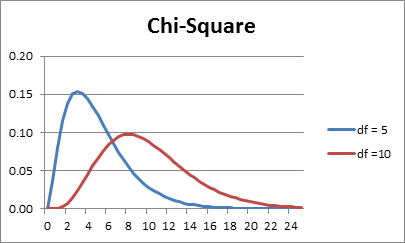# Chi-square Distribution

Definition 1: The chi-square distribution with k degrees of freedom, abbreviated χ2(k), has probability density functionk does not have to be an integer and can be any positive real number.

Click here for more technical details about the chi-square distribution, including proofs of some of the propositions described below. Except for the proof of Corollary 2 knowledge of calculus will be required.

Observation: The chi-square distribution is the gamma distribution where α = k/2 and β = 2.

Property 1: The χ2(k) distribution has mean k and variance 2k

Observation: The key statistical properties of the chi-square distribution are:

• Mean = k
• Median ≈ k(1–2/(9k))^3
• Mode = max (k – 1, 0)
• Range = [0.∞)
• Variance = 2k
• Skewness =$sqrt{8/k}$
• Kurtosis = 12/k

The following are the graphs of the pdf with degrees of freedom df = 5 and 10. As df grows larger the fat part of the curve shifts to the right and becomes more like the graph of a normal distribution.Figure 1 – Chart of chi-square distributions

Theorem 1: Suppose x has standard normal distribution N(0, 1) and let x1, …, xkbe k independent sample values of x, then the random variablehas a chi-square distribution χ2(k).

Corollary 1:

1. If x has distribution N(0, 1) then x2 has distribution χ2(1)
2. If x ~ N(μ, σ) and z = (x–μ)/σ then over repeated samples z2 has distribution χ2(1)
3. If x1, …, xkare independent observations from a normal population with normal distribution N(μ,σ) and for each iz = (x–μ)/σ , then the following random variable has a χ2(k) distributionProperty 2: If x and y are independent and x has distribution χ2(m) and y has distribution χ2(n), then x + y has distribution χ2(m + n)

Theorem 2: If x is drawn from a normally distributed population N(μ,σ) then for samples of size n the sample variance s2 has distributionCorollary 2s2 is an unbiased, consistent estimator of the population variance

Corollary 3: If x is drawn from a normally distributed population N(μ, σ), then for samples of size n the random variable$frac{(n-1)s^2}{sigma^2}$ has a χ2(n–1), distribution

Property 3: The mean of the sample variance s2 is σ2 and the variance is$frac{2sigma^4}{n-1}$

Proof: This can be seen from the proof of Corollary 2.

Excel Functions: Excel provides the following functions:

CHIDIST(x, df) = the probability that the chi-square distribution with df degrees of freedom is ≥ x; i.e. 1 – F(x) where F is the cumulative chi-square distribution function.

CHIINV(α, df) = the value x such that CHIDIST(x, df) = 1 – α; i.e. the value x such that the right tail of the chi-square distribution with area α occurs at x. This means that F(x) = 1 – α, where F is the cumulative chi-square distribution function.

With Excel 2010/2013 there are a number of new functions (CHISQ.DIST, CHISQ.INV, CHISQ.DIST.RT and CHISQ.INV.RT) that provide equivalent functionality to CHIDIST and CHIINV, but whose syntax is more consistent with other distribution functions. These functions are described in Built-in Statistical Functions.

In Excel 2010 CHISQ.DIST(x, df, TRUE) is the cumulative distribution function for the chi-square distribution with df degrees of freedom, i.e. 1 – CHIDIST(x, df), and CHISQ.DIST(xdf, FALSE) is the pdf for the chi-square distribution.

Real Statistics Functions: The Real Statistics Resource Pack provides the following functions.

CHISQ_DIST(x, df, cum) = GAMMA.DIST(x, df/2, 2, cum) = GAMMADIST(x, df/2, 2, cum)

CHISQ_INV(p, df) = GAMMA.INV(p, df/2, 2) = GAMMAINV(p, df/2, 2)

These functions provide better estimates of the chi-square distribution when df is not an integer. The first function is also useful in providing an estimate of the pdf for versions of Excel prior to Excel 2010, where CHISQ.DIST(x, df, FALSE) is not available.

The Real Statistics Resource also provides the following functions:

CHISQ_DIST_RT(x, df) = 1 – CHISQ_DIST(x, df, TRUE)

CHISQ_INV_RT(p, df) = 1 – CHISQ_INV(p, df)

Example 1: Suppose we take samples of size 10 from a population with normal distribution N(0, 2). Find the mean and variance of the sample distribution of s2.Statistics with R for Business Analysts – Normal Distribution

# Personal Career & Learning Guide for Data Analyst, Data Engineer and Data Scientist

## Applied Machine Learning & Data Science Projects and Coding Recipes for Beginners

A list of FREE programming examples together with eTutorials & eBooks @ SETScholars

# Projects and Coding Recipes, eTutorials and eBooks: The best All-in-One resources for Data Analyst, Data Scientist, Machine Learning Engineer and Software Developer

Topics included: Classification, Clustering, Regression, Forecasting, Algorithms, Data Structures, Data Analytics & Data Science, Deep Learning, Machine Learning, Programming Languages and Software Tools & Packages.
(Discount is valid for limited time only)`Disclaimer: The information and code presented within this recipe/tutorial is only for educational and coaching purposes for beginners and developers. Anyone can practice and apply the recipe/tutorial presented here, but the reader is taking full responsibility for his/her actions. The author (content curator) of this recipe (code / program) has made every effort to ensure the accuracy of the information was correct at time of publication. The author (content curator) does not assume and hereby disclaims any liability to any party for any loss, damage, or disruption caused by errors or omissions, whether such errors or omissions result from accident, negligence, or any other cause. The information presented here could also be found in public knowledge domains.`

# Learn by Coding: v-Tutorials on Applied Machine Learning and Data Science for Beginners

Please do not waste your valuable time by watching videos, rather use end-to-end (Python and R) recipes from Professional Data Scientists to practice coding, and land the most demandable jobs in the fields of Predictive analytics & AI (Machine Learning and Data Science).

The objective is to guide the developers & analysts to “Learn how to Code” for Applied AI using end-to-end coding solutions, and unlock the world of opportunities!